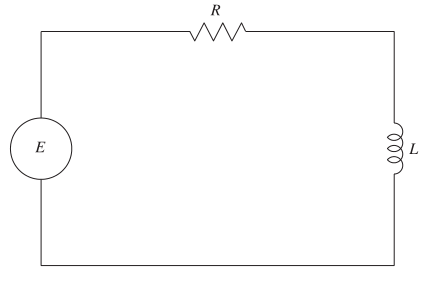Chapter 6.1, Problem 91E

Chapter
Section
Textbook Problem

Electric Circuit The diagram shows a simple electric circuit consisting of a power source, aresistor, and an inductor.A model of the current I, in amperes (A) at time t is given by the first-order differential equation L d I d t + R I = E ( t ) where E(t) is the voltage (V) produced by the power source, R is the resistance, in ohms Ω andis the inductance, in henrys (H). Suppose the electric circuit consists of a 24-V power source, a12- resistor, and a 4-H inductor.(a) Sketch a slope field for the differential equation.(b) What is the limiting value of the current? Explain.

a)

To determine

To Graph: A slope field for the given differential equation.

Explanation

Given:

LdIdt+RI=E(t), where E(t) =24V, R =12Ω and L =4H inductor.

Graph:

Using the differential equation LdIdt+RI=E(t) …… (1)

It is found that

4dIdt+12I=24dIdt=63I

The slope field is plotted below using a graphing utility desmos tool

b)

To determine
The limiting value of the current.

Still sussing out bartleby?

Check out a sample textbook solution.

See a sample solution

The Solution to Your Study Problems

Bartleby provides explanations to thousands of textbook problems written by our experts, many with advanced degrees!

Get Started

Intervals Express each set in interval notation. 60. (a) (b)

Precalculus: Mathematics for Calculus (Standalone Book)

Convert the expressions in Exercises 8596 radical form. 34(1x)5/2

Finite Mathematics and Applied Calculus (MindTap Course List)

Find the mean for the following set of scores: 2, 7, 9, 4, 5, 3, 0, 6

Essentials of Statistics for The Behavioral Sciences (MindTap Course List)

Given: m13=70 Find: m3

Elementary Geometry for College Students